Home Tech & ScienceArtificial Intelligence (AI) TensorFlow Tutorial for Beginners

# TensorFlow Tutorial for Beginners

0 comment

TensorFlow is an open-source software library for dataflow programming, which is used for machine learning applications such as neural networks. It was developed by the Google Brain team for internal Google use. It allows developers to create dataflow graphs for machine learning algorithms, and the library provides tools for visualization and debugging. It is used by companies such as Google, Microsoft, Uber, Intel, and Twitter.

In this tutorial, we will cover the basics of TensorFlow, including how to install it, how to build a simple machine learning model, and how to train and evaluate the model.

Installation

To install TensorFlow, you will need to have Python 3.5 or higher installed on your system. You can then install TensorFlow using pip, the Python package manager. Open a terminal and enter the following command: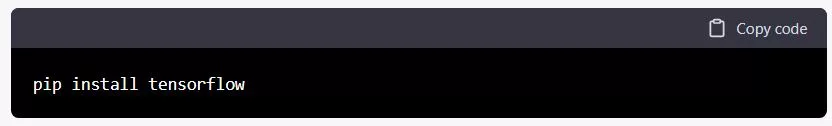Alternatively, you can install TensorFlow with conda, the package manager for the Anaconda Python distribution. Open a terminal and enter the following command: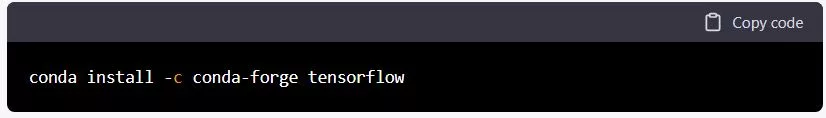Building a Simple Model

TensorFlow uses a dataflow programming model, which allows users to build complex machine learning models by building a graph of operations. The basic building block in TensorFlow is the Tensor. A Tensor is a multi-dimensional array of data, similar to a matrix.

To build a simple model in TensorFlow, we will start by importing the library and creating a Tensor. Here is an example of how to create a 2×2 tensor with all elements set to 1:This will print the following output: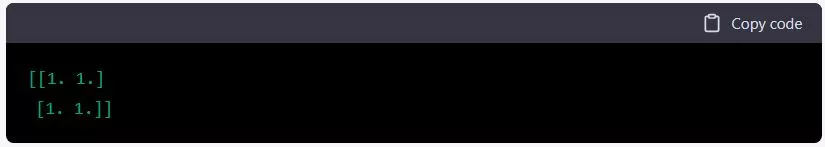We can also perform operations on tensors, such as element-wise addition or multiplication. For example, here is how to add two tensors element-wise: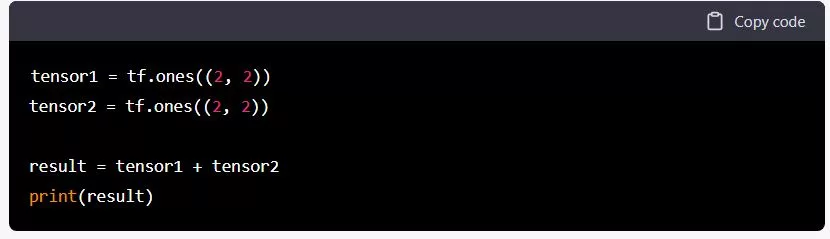This will print the following output: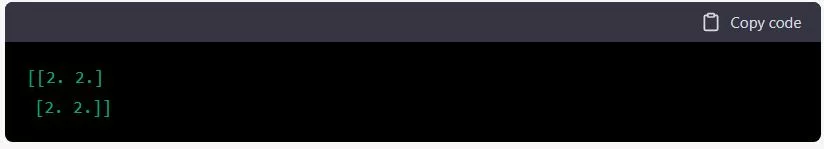We can also use TensorFlow to perform more complex operations, such as matrix multiplication. For example, here is how to multiply two matrices: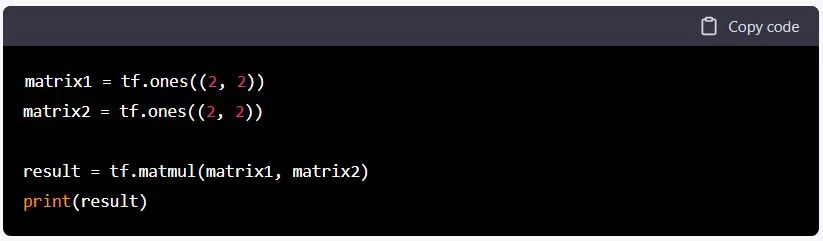This will print the following output: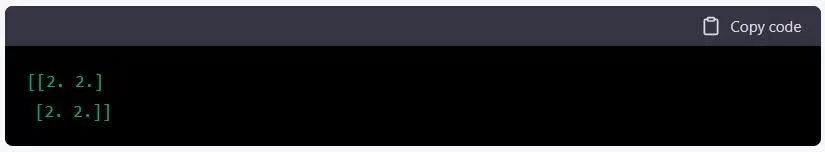Training and Evaluating a Model
To train and evaluate a machine learning model in TensorFlow, we will need to define a loss function and an optimizer. The loss function measures how well the model is performing, and the optimizer determines how the model should be updated based on the loss function.

For example, here is how to define a linear regression model in TensorFlow: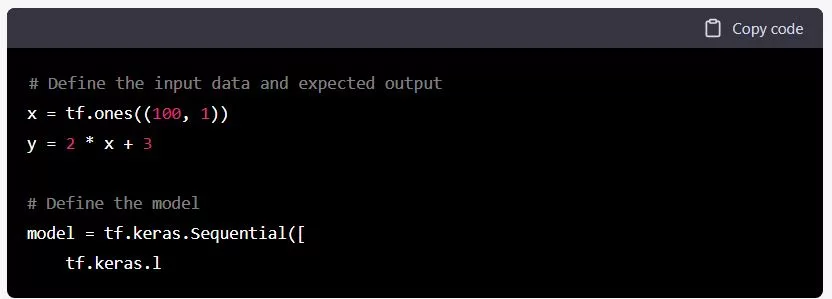To train the model, we will need to define an optimizer and a loss function. For this example, we will use the mean squared error as the loss function and the Adam optimizer.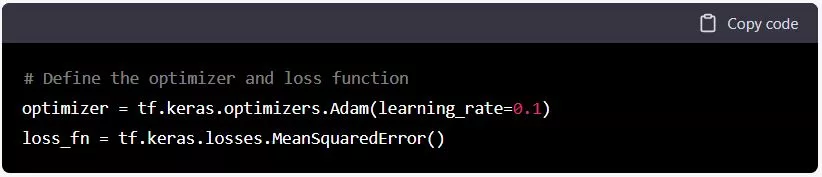Next, we will define a function to compute the forward pass of the model and calculate the loss.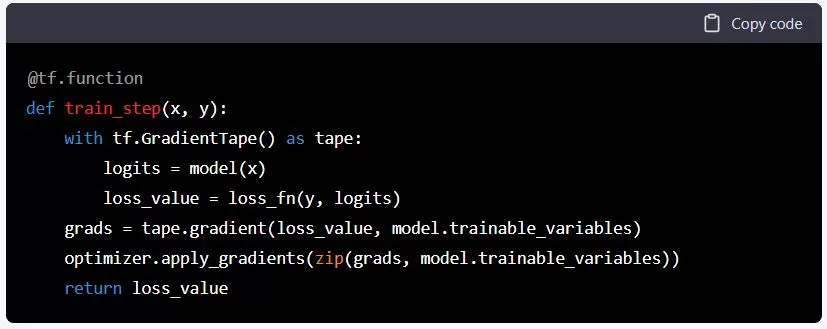Finally, we can use a loop to train the model for a number of epochs. An epoch is a complete pass through the training data.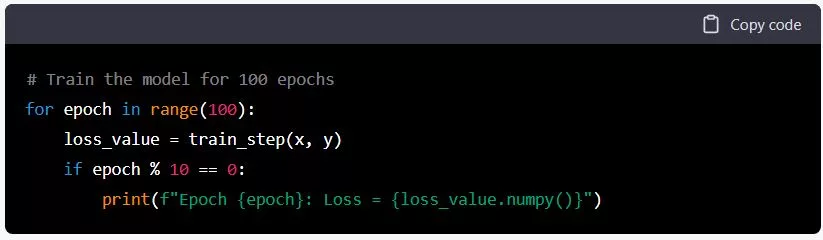To evaluate the model, we can use the evaluate method of the model. This method takes a dataset as input and returns the loss and any additional metrics we have defined.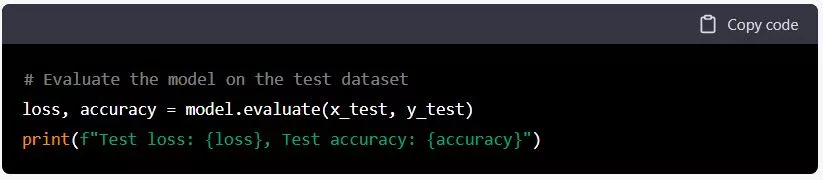That’s it! You now know the basics of how to build, train, and evaluate a machine learning model using TensorFlow. There are many more advanced features and techniques available in TensorFlow, but this should give you a good foundation to build upon.

(Visited 28 times, 1 visits today)
You Might Also Like

#### You may also likeBreaking News on Health, Science, Politic, Science, Entertainment!